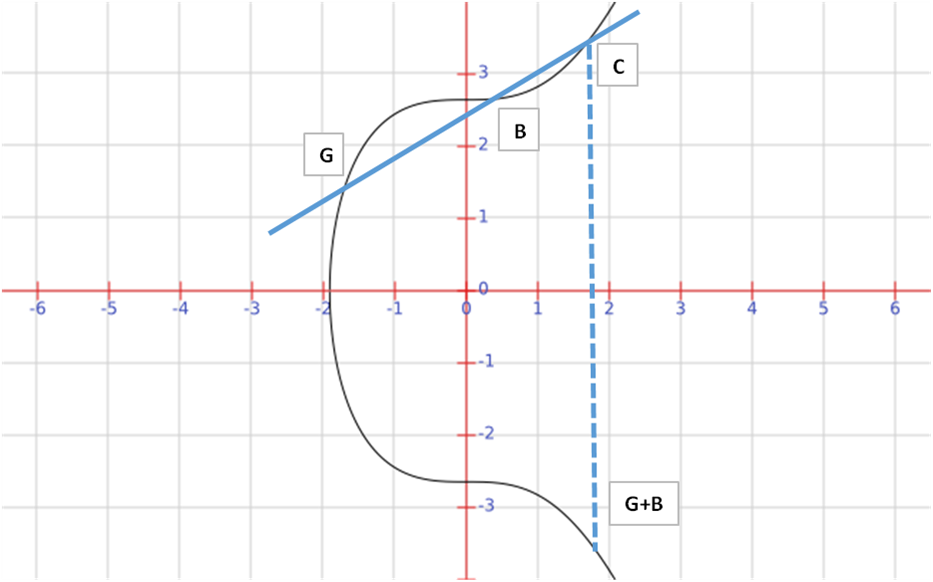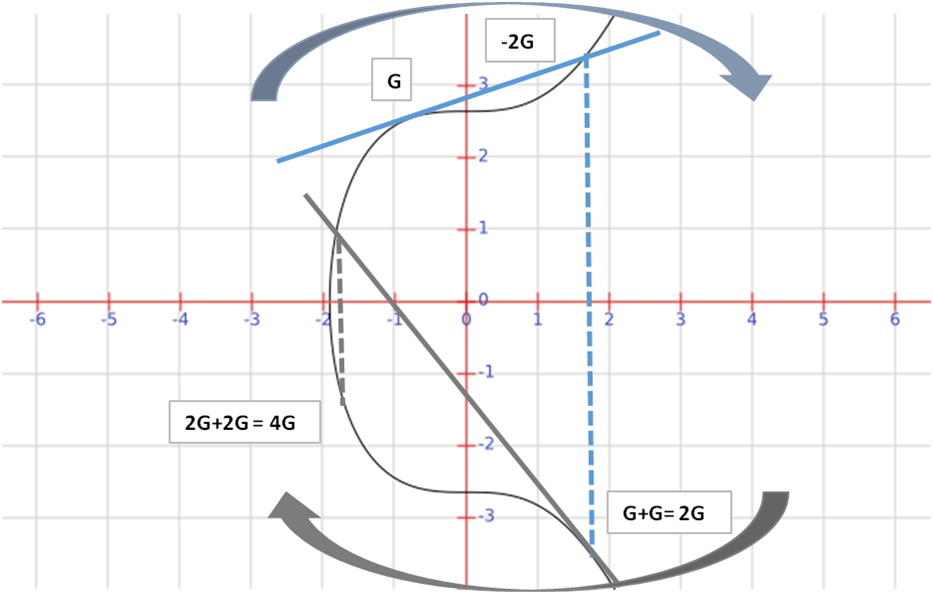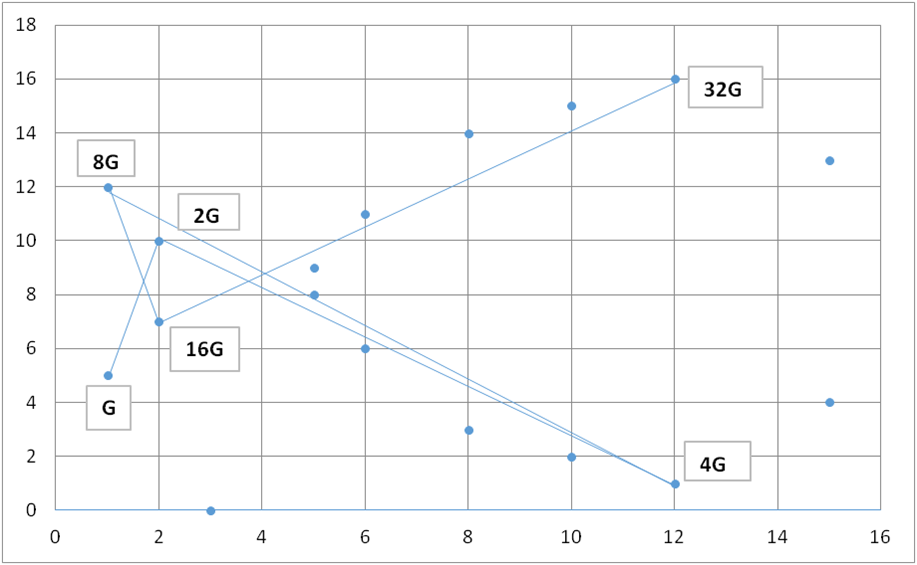# What is Elliptic Curve Cryptography?

12 Feb 2015Elliptic curve cryptography is a branch of mathematics that deals with curves or functions that take the format

y2=x3+ax+b

These curves have some properties that are of interest and use in cryptography – where we define the addition of points as the reflection in the x axis of the third point that intersects the curve.

Firstly we calculate the equation of the line G to B and find where it intersects the function and multiply the (y) coordinate by (-1).  This is a very simple geometric and algebraic calculation when the two points are known.What happens though if we want to add two points which  are the same – for example we want to add G + G = 2G. This is similar to standard calculus methods of calculating derivatives and we end up just taking tangent to the curve at the point and find where it intersects the curve and again flip it in the x – axis.In fact, as shown in the picture above we can repeat the process and repeat the process. So to get 4G we can take the point 2G and add it to itself again to find 4G – again this is done by taking the tangent of the curve at 2G and finding where it intersects the function. This also shows you how you can quickly perform multiple multiplications quickly - to calculate 4G takes two calculations instead of 3 and can be extended to 32G taking 5 steps instead of 33.

The Bitcoin curve is based secp256k1 curve based on modular mathematics and takes the form below:

y2=x3+7 mod n where n=1.158x1077

This introduces a new form of mathematics of group field theory or modular arithmetic. Even though it sounds intimidating it’s actually quite simple and straight forward and we are all very familiar with it in the form of clocks! As clocks measure in 12 hour sets then 12 hours from any point is just the same point – and in Bitcoin this field (or clock) is 1.158x10^77 units. You can imagine the chart wrapping round on itself.

Another point is that when defined in this way as a field on prime order n the curve no longer takes real numbers (i.e. decimals) which is very useful in computing as you don’t get decimals and error codes. In fact the curve instead of being smooth becomes a series of dots. The figure below shows a curve of finite field of prime order 17.In fact multiplication and addition in this space obey the same principles as normal elliptic curves just with a bit of changing from modular arithmetic. You can still get the same results as with elliptic curves over real numbers when you add points together which looks like below – although the simple geometry breaks down and it looks fully chaotic and random.This is how Bitcoin creates Public Keys from private keys. The equation below is the transformation of a private key to a public key.

Public_K= G x Private_K

In this case the Public_K is a point in the secp2561k curve with both x and y coordinates and G is the generator point. The generator point is the same for everyone and is just an arbitrarily defined point.

If the Private_K is 32 then the multiplication of Private_K and the point G(x,y) is simply 32G is:

Private_K x G=32G=G+G+G+G…..G

This is where the discrete logarithm problem comes in and is what gives public and private keys their security and one way trajectory.